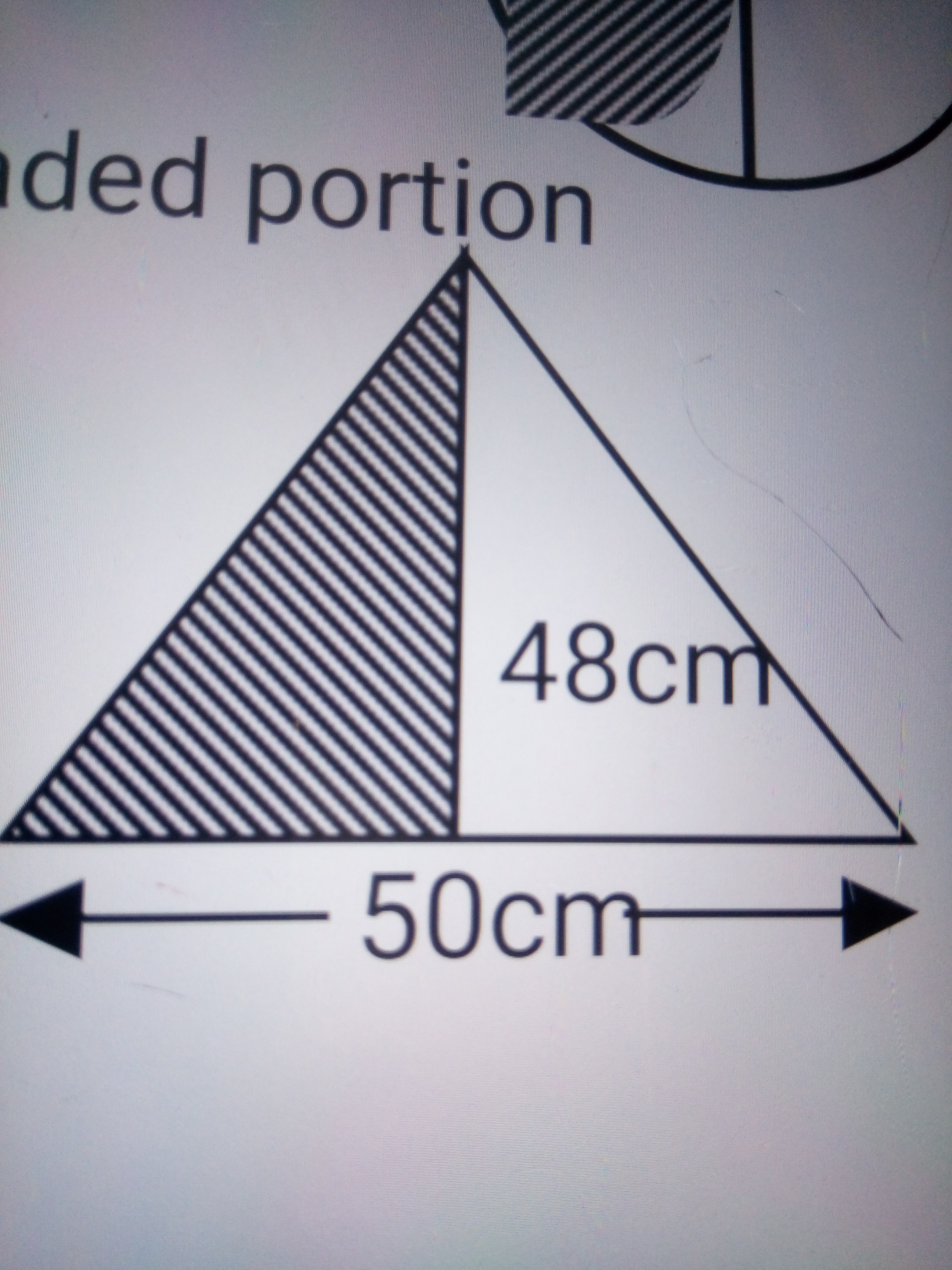# Primary 6 Second Term Examinations Mathematics

SECOND TERM EXAMINATION 2020
CLASS: PRIMARY 6 SUBJECT: MATHEMATICS
NAME:……………………………………………………………………………………
Instructions: Answer the following questions
Part A: Objectives
1.) What is the difference between 1¾ of ¼ and ½ (a) ½ (b) 3/7 (c) 3/8 (d) ¼
2.) What percentage of N1.50 is left after giving out 105k
(a) 95% (b) 55% (c) 45% (d) 30%
3.) What is 24% of N250 (a) N30 (b) N48 (c) N50 (d) N60
4.) A trader sold an article for N150 and made a profit of 20%. What was the cost price?
(a) N 20 (b) N120 (c) N125 (d) N150

5.) Which of the following is a prime number (a) 4 (b) 5 (c) 6 (d) 8
6.) Calculate the area of the shaded part of the diagram below
(a) 120cm2 (b) 22cm2 (c) 60cm2 (d) 240cm2

7.) Write 20110110 in words (a) twelve billion, twenty thousand and eleven million
(b) twenty million, one hundred and ten thousand, one hundred and ten (c) twenty thousand, eleven million and eleven ten (d) twenty billion, eleven million and hundred ten

8.) Express 1463 in Roman figures (a) DCXLIII (b) MCDLXIII (c) MDCXIII (d) MMCCXXIII
9.) Take away 298433 from 656500 (a) 26657 (b) 35087 (c) 358067 (d) 384087
10.) If five apples cost N250. Calculate the cost of forty-five apples
(a) N45250 (b) N2250 (c) N12455 (d) N3555
11.) Subtract 0.07 from 1.1 (a) 1.3 (b) 0.13 (c) 1.03 (d) 1.07
12.) Express 514 in roman figures (a) CCXLV (b) DXIV (c) DXLV (d) XDIV
13.) Find the smallest number that can be divided by 6, 5 and 4 (a) 30 (b) 40 (c) 60 (d) 120
14.) Express 25k as a percentage of N5.00 (a) 50% (b) 25% (c) 5% (d) 15%
15.) Find the value of the angle that is marked x
(a) 200 (b) 1300 (c) 1200 (d) 1100

16.) Write DXIV in Arabic numeral (a) 545 (b) 514 (c) 404 (d) 440
17.) Calculate the HCF of 13 and 39 (a) 3 (b) 1 (c) 13 (d) 39
18.) Which of the following is a prime factor (a) 4 (b) 5 (c) 6 (d) 8
19.) How many digit are there in one million (a) one (b) six (c) seven (d) nine
20.) Find the L.C.M of 4 and 8 (a) 4 (b) 2 (c) 8 (d) 16

Part B: Theory
Show all workings
1.) Add up 5.976, 164.8 and 98.33
b.) Find the difference between 818.07 and 36.1984

2.) Multiply 32.4 by 1.26
b.) Find the product of 0.006 and 1.011

3.) Calculate the area of the shaded portionb.) Calculate the area of the shaded portion4.) Write out all factors of 36
b.) Write out prime factors of 30

5.) Calculate the simple interest of N500 for 5yrs at the rate of 5% per annum
b.) Multiply the L.C. M of 8 and 12 by its HCF.